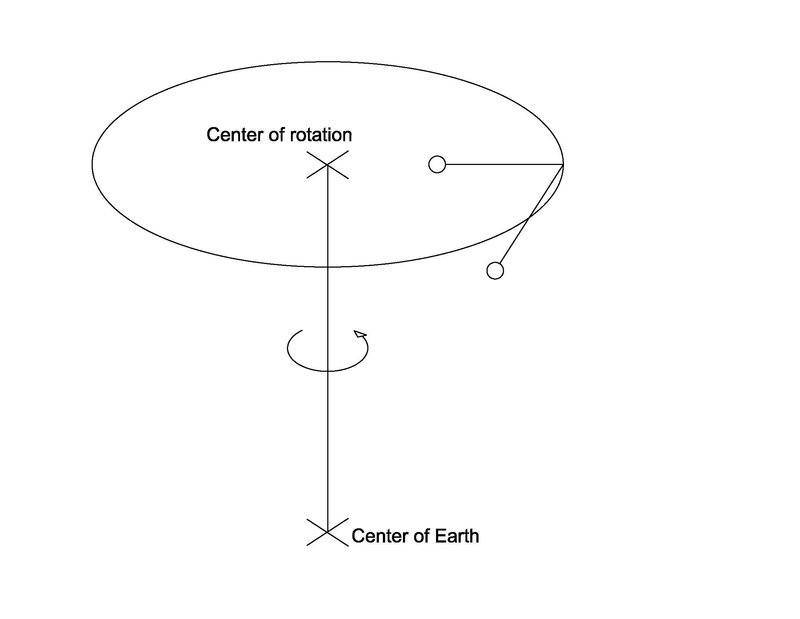# Deviation of Plumb Bob In Uniform Circular Motion

mopit_011
Homework Statement:
Because of the rotation of the Earth, a plumb bob may not hang exactly along the direction of the Earth’s gravitational force on the plumb bob but may deviate slightly from that direction. (a) Show that the deflection theta in radians at a point is equivalent to ##\theta = (2\pi^2R/gT^2)(\sin 2L)## where L is the latitude at the point, R is the radius, and T is the period of the Earth’s rotation.
Relevant Equations:
Net force in uniform circular motion = (mv^2/r).
I started by making my coordinate system so that the x-axis aligned with the radius of the circle at a certain latitude L and the positive direction was facing away from the center of the circle, and the y-axis was parallel to the vertical axis of the Earth. Then, I wrote the equations for the net forces for both axes. Here are the equations I wrote:

(1) ##F_{x}## = ##T \cos (L + \theta) ## ##- mg## ##\cos(L)## ##= (mv^2/R \cos L)## where R is the radius of the Earth.
(2) ##F_{y} = T \sin (L + \theta) - mg \sin (L)##.

Then I solved for T from the second equation and plugged it into the first equation.
When I tried to solve for theta, I was unable to show the desired deflection angle was correct. Are my equations wrong or am I missing something else? Thank you!

•Delta2

Homework Helper
Gold Member
Your plumb bob, and its centripetal acceleration vector, are rotating around a circle that is not coplanar with the geometrical center of the Earth and the gravity acceleration vector.
A representation of those vectors should give you the deflection theta in radians.Last edited:
•Delta2
Homework Helper
Gold Member
2022 Award
I was unable to show the desired deflection angle was correct.
Please post that working. In particular, how did you replace v?

Homework Helper
Gold Member
Hmm, a rigorous treatment of this requires the concept of Coriolis force.

Homework Helper
Gold Member
2022 Award
Hmm, a rigorous treatment of this requires the concept of Coriolis force.
Only if working in a non inertial frame. Anyway, this is static in the obvious non inertial frame, so no Coriolis.

•Delta2
Homework Helper
Gold Member
BTW is a bit of unfortunate that the OP chose the symbol ##T## for the tension of the string which the problem statement uses for the period of the earth.

Hmm, so what is left here is replacing ##v=\frac{2\pi R}{T}## (T the period of Earth not the tension) and doing some algebra and trigonometry.

Staff Emeritus
Homework Helper
Gold Member
Let's wait and see what OP has to say in response - especially in response to @haruspex .

mopit_011
Please post that working. In particular, how did you replace v?

I replaced v with ##(2\pi)(R \cos L)/T## and ##mg\sin L/ \sin(L +\theta)## with T (the tension) and substituted them into the first equation to get the following equation.

## (mg \sin L/\sin (L+ \theta))## ##- mg \cos L = -m ((2\pi R \cos L)/T)^2/R \cos L##

Then, I canceled out m from both sides in the equation and then rewrote the equation as ##(g \sin L/\tan (L + \theta)) - g \cos L = -4 \pi^2 R \cos L/T^2##

Next, I added ##g \cos L## to both sides of the equation and divided by ##g \sin L## to get
##1/\tan (L + \theta)## ##= (-4\pi^2 R \cos L + T^2g\cos L)/T^2(g\sin L)##.

This simplifies to ##1/\tan (L + \theta)## ##= -4\pi^2R/T^2g\tan(L) + (1/g \tan L)##.

I'm unsure of how to further solve for theta from this.

•Delta2
Homework Helper
Gold Member
2022 Award
I replaced v with ##(2\pi)(R \cos L)/T## and ##mg\sin L/ \sin(L +\theta)## with T (the tension) and substituted them into the first equation to get the following equation.

## (mg \sin L/\sin (L+ \theta))## ##- mg \cos L = -m ((2\pi R \cos L)/T)^2/R \cos L##

Then, I canceled out m from both sides in the equation and then rewrote the equation as ##(g \sin L/\tan (L + \theta)) - g \cos L = -4 \pi^2 R \cos L/T^2##

Next, I added ##g \cos L## to both sides of the equation and divided by ##g \sin L## to get
##1/\tan (L + \theta)## ##= (-4\pi^2 R \cos L + T^2g\cos L)/T^2(g\sin L)##.

This simplifies to ##1/\tan (L + \theta)## ##= -4\pi^2R/T^2g\tan(L) + (1/g \tan L)##.

I'm unsure of how to further solve for theta from this.
Your last equation is dimensionally inconsistent. There is a g in the denominator in the last term that should have cancelled.
The next step is to use an approximation for small ##\theta##.

•Delta2
Homework Helper
Gold Member
Your last equation is dimensionally inconsistent. There is a g in the denominator in the last term that should have cancelled.
The next step is to use an approximation for small ##\theta##.

What approximation do you have in mind? to approximate ##\tan(L+\theta)=\frac{\tan L+\tan\theta}{1-\tan L \tan\theta}## by setting the numerator equal to ##\tan L##? Tried that but ... didnt work as I expected

••